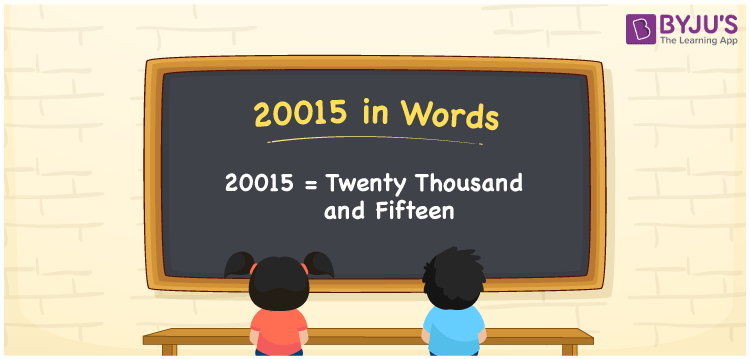# 20015 in Words

We can write 20015 in words as Twenty thousand and fifteen. We can efficiently transform the number 20015 into words using a place value chart. It is important to note that 20015 is a cardinal number since it denotes a specific quantity or amount. Furthermore, Twenty thousand and fifteen is the number name or word form of 20015.

 20015 in words Twenty thousand and fifteen Twenty thousand and fifteen in Numbers 20015

## 20015 in English words

Generally, we write numbers in words using the English alphabet. So, we spell 20015 in English as “Twenty thousand and fifteen”.## How to Write 20015 in Words?

Let us derive the number 20015 in words using a five-column place value chart given below.

 Ten thousands Thousands Hundreds Tens Ones 2 0 0 1 5

Here, ones = 5, tens = 1, hundreds = 0, thousands = 0, ten thousands = 2

These numbers can be expanded as:

2 × Ten thousand + 0 × Thousand + 0 × Hundred + 1 × Ten + 5 × One

= 2 × 10000 + 1 × 10 + 5 × 1

= 20000 + 10 + 5

= 20000 + 15

= Twenty thousand + Fifteen

= Twenty thousand and fifteen

Therefore, 20015 in words = Twenty thousand and fifteen.

As we know, 20015 is a natural number that is the successor of 20014 and the predecessor of 20016.

20015 in words – Twenty thousand and fifteen

Is 20015 an even number? – No

Is 20015 an odd number? – Yes

Is 20015 a perfect square number? – No

Is 20015 a perfect cube number? – No

Is 20015 a prime number? – No

Is 20015 a composite number? – Yes

## Frequently Asked Questions on 20015 in Words

Q1

### Write the number 20015 in words.

We can write the number 20015 in words as Twenty thousand and fifteen.
Q2

### How to write Rs. 20015 in words on a cheque?

On a cheque, we generally write Rs. 20015 in words as “Twenty thousand and fifteen rupees only”.
Q3

### What is the spelling of 20015?

The spelling of 20015 in English words is Twenty thousand and fifteen.